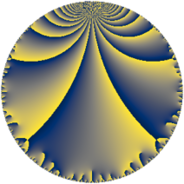# Properties

 Label 75.6.gLevel $75$ Weight $6$ Character orbit 75.g Rep. character $\chi_{75}(16,\cdot)$ Character field $\Q(\zeta_{5})$ Dimension $104$ Newform subspaces $2$ Sturm bound $60$ Trace bound $2$

# Learn more about

## Defining parameters

 Level: $$N$$ $$=$$ $$75 = 3 \cdot 5^{2}$$ Weight: $$k$$ $$=$$ $$6$$ Character orbit: $$[\chi]$$ $$=$$ 75.g (of order $$5$$ and degree $$4$$) Character conductor: $$\operatorname{cond}(\chi)$$ $$=$$ $$25$$ Character field: $$\Q(\zeta_{5})$$ Newform subspaces: $$2$$ Sturm bound: $$60$$ Trace bound: $$2$$ Distinguishing $$T_p$$: $$2$$

## Dimensions

The following table gives the dimensions of various subspaces of $$M_{6}(75, [\chi])$$.

Total New Old
Modular forms 208 104 104
Cusp forms 192 104 88
Eisenstein series 16 0 16

## Trace form

 $$104q + 8q^{2} - 448q^{4} - 6q^{5} - 72q^{6} + 312q^{7} + 1122q^{8} - 2106q^{9} + O(q^{10})$$ $$104q + 8q^{2} - 448q^{4} - 6q^{5} - 72q^{6} + 312q^{7} + 1122q^{8} - 2106q^{9} + 186q^{10} - 474q^{11} + 792q^{12} - 492q^{13} + 2748q^{14} + 396q^{15} - 12020q^{16} - 2510q^{17} - 2592q^{18} - 5522q^{19} - 4624q^{20} - 1764q^{21} + 3330q^{22} - 2124q^{23} + 13824q^{24} - 2776q^{25} + 3428q^{26} - 8076q^{28} - 18404q^{29} - 11916q^{30} - 1566q^{31} - 47508q^{32} + 11934q^{33} - 12376q^{34} + 31650q^{35} - 36288q^{36} + 34532q^{37} + 5954q^{38} - 12168q^{39} - 27068q^{40} + 15774q^{41} + 11916q^{42} - 90688q^{43} - 10814q^{44} - 486q^{45} - 22956q^{46} + 104944q^{47} + 70128q^{48} + 356720q^{49} + 376q^{50} + 83232q^{51} - 68900q^{52} + 51336q^{53} - 5832q^{54} - 4346q^{55} - 116100q^{56} - 9216q^{57} - 1262q^{58} - 97428q^{59} - 5886q^{60} - 53660q^{61} + 308014q^{62} - 22518q^{63} - 77062q^{64} + 291552q^{65} - 118296q^{66} - 204320q^{67} - 247784q^{68} - 73332q^{69} + 115060q^{70} - 155672q^{71} + 90882q^{72} + 205896q^{73} + 92988q^{74} + 67176q^{75} + 464032q^{76} - 194536q^{77} + 168120q^{78} - 112020q^{79} - 98384q^{80} - 170586q^{81} + 326904q^{82} + 360614q^{83} - 61128q^{84} - 752802q^{85} + 295956q^{86} - 398232q^{87} - 974738q^{88} - 340932q^{89} - 201204q^{90} + 79494q^{91} - 955258q^{92} + 49608q^{93} + 63522q^{94} + 17136q^{95} - 344844q^{96} + 600740q^{97} + 1297452q^{98} + 25596q^{99} + O(q^{100})$$

## Decomposition of $$S_{6}^{\mathrm{new}}(75, [\chi])$$ into newform subspaces

Label Dim. $$A$$ Field CM Traces $q$-expansion
$$a_2$$ $$a_3$$ $$a_5$$ $$a_7$$
75.6.g.a $$52$$ $$12.029$$ None $$0$$ $$-117$$ $$-36$$ $$548$$
75.6.g.b $$52$$ $$12.029$$ None $$8$$ $$117$$ $$30$$ $$-236$$

## Decomposition of $$S_{6}^{\mathrm{old}}(75, [\chi])$$ into lower level spaces

$$S_{6}^{\mathrm{old}}(75, [\chi]) \cong$$ $$S_{6}^{\mathrm{new}}(25, [\chi])$$$$^{\oplus 2}$$